If you have dreams of becoming a designer, you'll need to know your measurements!

# Measurements - Inches, Half Inches, Quarter Inches and Eighth Inches (Add and Subtract)

This Math quiz is called 'Measurements - Inches, Half Inches, Quarter Inches and Eighth Inches (Add and Subtract)' and it has been written by teachers to help you if you are studying the subject at middle school. Playing educational quizzes is a fabulous way to learn if you are in the 6th, 7th or 8th grade - aged 11 to 14.

It costs only \$12.50 per month to play this quiz and over 3,500 others that help you with your school work. You can subscribe on the page at Join Us

For several years now you have been learning about how to measure things such as how tall are you or the length of your room and/or how wide or how long a table is. This can all be determined by using a ruler or measuring tape. A ruler generally is the length of 12 inches.

You also learned that 12 inches is equal to 1 foot. Finally, you learned that 3 feet equals 1 yard. This all might seem second nature to you now but it is always important to review, even the basics, before heading into a new area of math such as the metric system. So this quiz will revisit as a refresher the way to add, subtract, multiply and divide measurements.

To make it a little more challenging, we will go beyond just the inch, foot and yard because, although these measurements are very good, what happens when something is not equal to a perfect inch or a perfect foot or even a perfect yard?

The solution is that there are measurements that are smaller than an inch. These measurements include the half inch where 2 half inches are equal to an inch, or four quarter inches where 4 quarters are equal to an inch and the eighth inch where 8 eighth inches are equal to an inch. In fact, we can even go smaller in our measurements on a ruler but for this quiz, we will stick to the inch, the half inch, the quarter inch and the eighth inch.

The math symbol for “inch” is " (almost looks like quotation marks) and the math symbol for a foot is ' which looks very much like an apostrophe.

For each of the following measurement problems, find the correct solution/answer.

Go straight to Quiz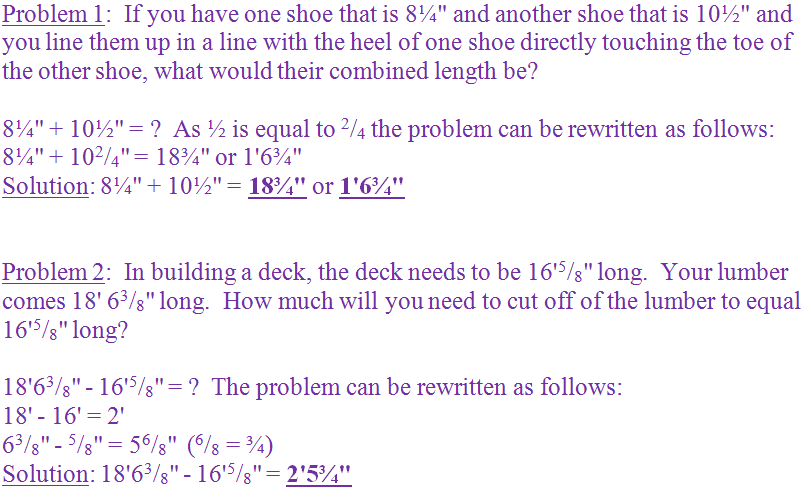Question 1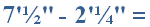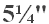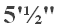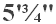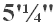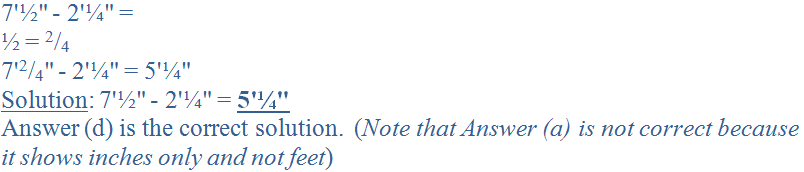Question 2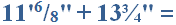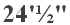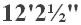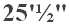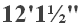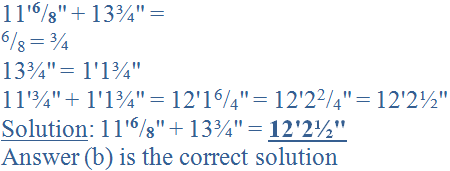Question 3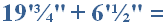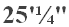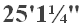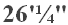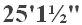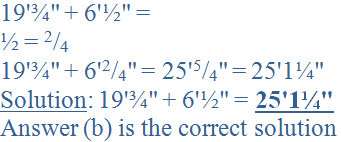Question 4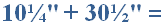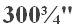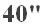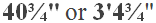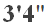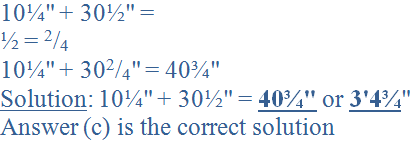Question 5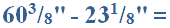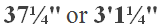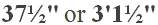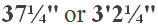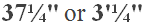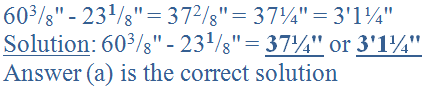Question 6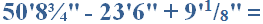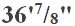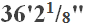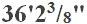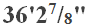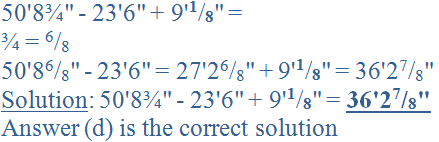Question 7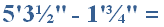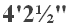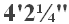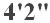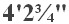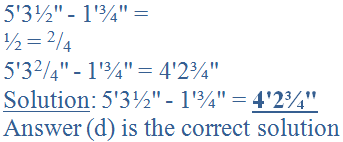Question 8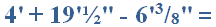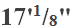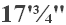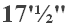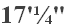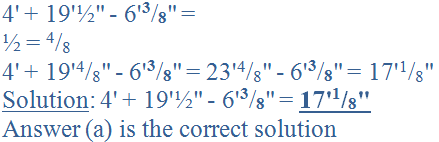Question 9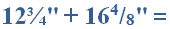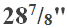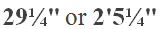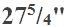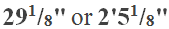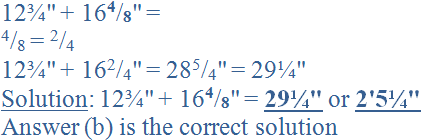Question 10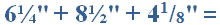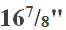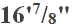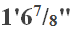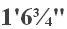Author:  Christine G. Broome